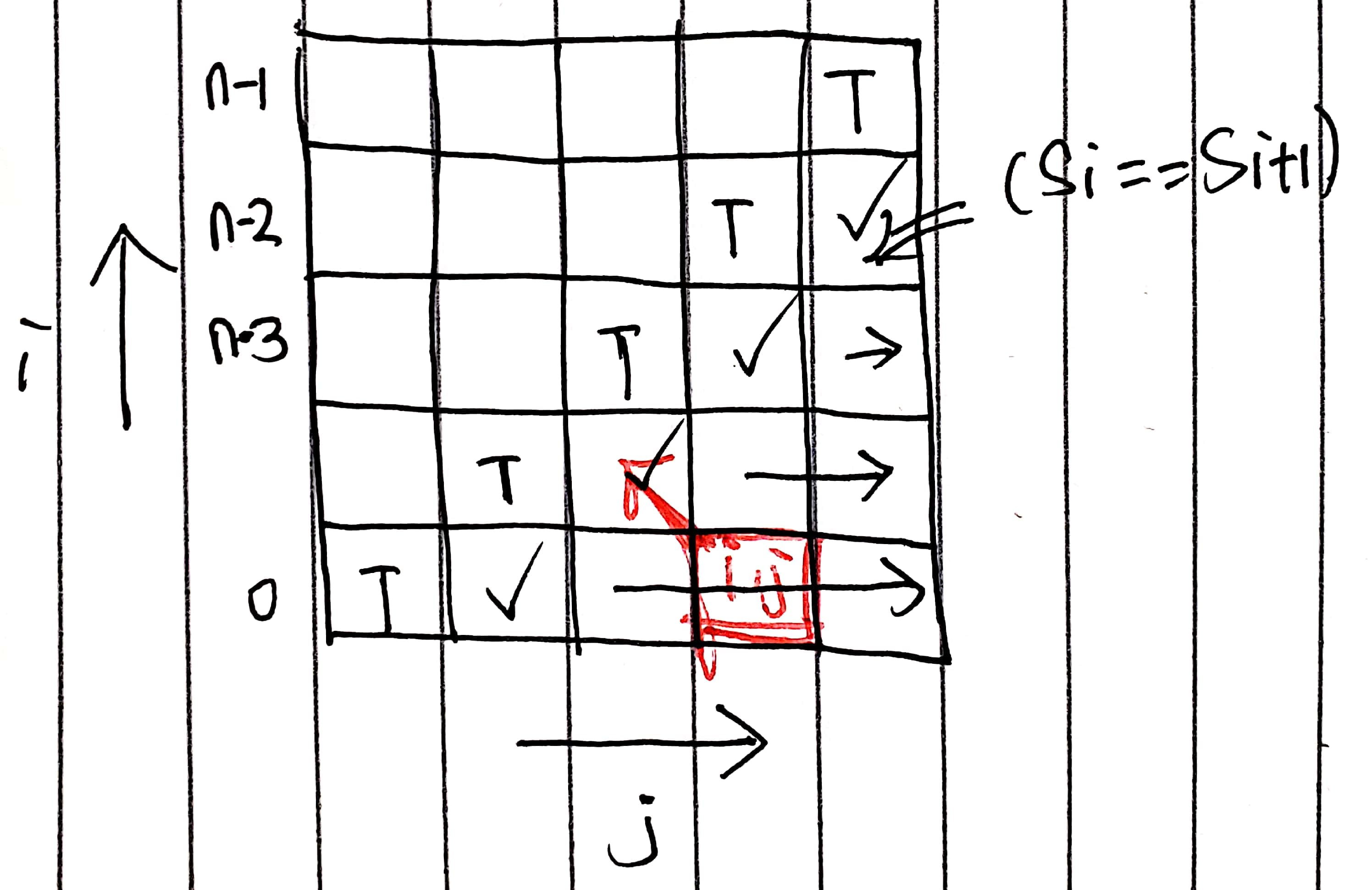# 5. Longest Palindromic Substring

Reference: LeetCode
Difficulty: Medium

## Problem

Given a string s, find the longest palindromic substring in s. You may assume that the maximum length of s is 1000.

Example:

## Analysis

### Brute-Force

For each character i, we examine all substrings starting from index i and check if they are palindromic.

Time: $O(N^3)$
Space: $O(1)$

### Expansion From the Center

Time: $O(N^2)$
Space: $O(N)$

### DP

Based on the brute-force solution, we can improve on it by avoiding unnecessary re-computation while validating palindromes. Consider the case ababa. If we already knew that bab is a palindrome, it is obvious that ababa must be a palindrome since the two end letters are the same.

So we can define dp[i][j] as true if the substring S(i, j) is a palindrome.

The recurrence is then obvious: dp[i][j] = (dp[i + 1][j - 1] && Si == Sj)

The base cases are: dp[i][i] = true, dp[i][i + 1] = (Si == Si+1).Note: Determining ranges of i and j would be easy if you pick a starting point in the graph to get some intuition. For example, in this case j starts from i + 2.

Time: $O(N^2)$
Space: $O(N^2)$

CommentJunhao Wang
a software engineering cat